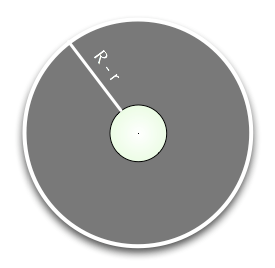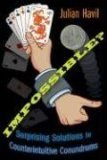# That’s impossible!

Imagine a rope that was just long enough to wrap tightly around the equator of a perfectly spherical earth. Now imagine that the length of that rope is increased by one meter and again wrapped around the earth, supported in a regular way, so as to form an annulus. Doing things in this way will form a certain gap between the earth and the extended rope. Now imagine that you repeat the process with a golf ball. How do the two gaps compare?

Most people who understand the problem correctly, will immediately assume that adding a single meter to the rope surrounding the huge spherical earth won’t create much of a gap, while adding a single meter to the rope around the golf ball, will create a large gap. In reality, the two gaps are identical. It’s counterintuitive, but it can be proven easily with elementary mathematics.

We know that:

$$C = 2 \pi r$$
$$C + 1 = 2 \pi R$$

Where C is the length of the rope around a given spherical object, C+1 is obviously the length of the longer (by one meter) rope, r is the radius of the object (e.g. the golf ball) and R the radius of the annulus. We can express the two equations above as such:

$$\displaystyle r = \frac{C}{2\pi}$$
$$\displaystyle R = \frac{C+1}{2\pi}$$

The width of the gap between the longer rope and the object that’s taken into consideration is R-r.Therefore:

$$\displaystyle R – r = \frac{C+1}{2\pi} – \frac{C}{2\pi} = \frac{1}{2\pi} \approx 0.159$$

The gap, as you can see, is constant at about 16 cm, and it doesn’t depend in any way on the size of the radius (r) of the object at hand. That means that the gap between the extended rope and the golf ball is the same as in the case of the spherical earth.Quite a surprising result, isn’t it? This exact example opens up the book Impossible?: Surprising Solutions to Counterintuitive Conundrums which I received as a media copy in the mail. So far it’s been a very enjoyable and easy read, chocked full of surprising paradoxes and results which common sense would have you deem (practically) impossible or counterintuitive. To make things even nicer, the math involved is not overly advanced, and anyone who grasped high school level math, should have no problem following this engaging book.

1.Enginerd May 11, 2008
2.Antonio Cangiano May 11, 2008
3.bob saget May 12, 2008
4.Adrian Mac August 14, 2008
5.sean October 3, 2008